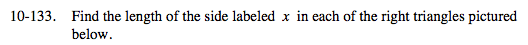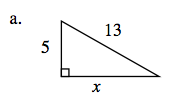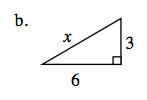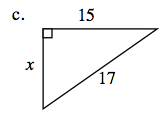### Home > MC2 > Chapter 10 > Lesson 10.2.5 > Problem10-133

10-133.Use the Pythagorean Theorem.(leg 1)² + (leg 2)² = (hypotenuse)²
Substitute the values that are given.

(5)² + (x)² = (13)²
25 + x² = 169

Solve by isolating x.

x² = 144
Take the square root of each side to get the answer.(leg 1)² + (leg 2)² = (hypotenuse)²
Substitute the values that are given.

(6)² + (3)² = (x
Now solve for x.See part (a).

8